Get instant live expert help with Excel or Google Sheets“My Excelchat expert helped me in less than 20 minutes, saving me what would have been 5 hours of work!”

#### Post your problem and you’ll get Expert help in seconds.

Your message must be at least 40 characters
Our professional Expert are available now. Your privacy is guaranteed.

# Using the COUNTIF Function of Excel’s Conditional Formatting

The usage of the COUNTIF & Conditional Formatting functions in Excel can yield useful and visually appealing results. This step by step tutorial will assist all levels of Excel users in creating dynamic conditional formats.

Our data-set shows the date and the type of vehicle rented. Now, we want to show the overall count of a type of vehicle with color coding.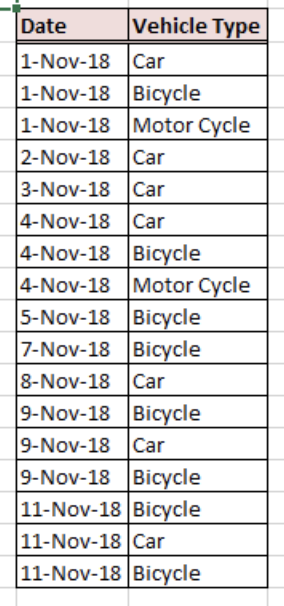Figure 1: Dataset containing Date and Vehicle Type

## Usage of the “COUNTIF” Excel Function

### Syntax of the COUNTIF Formula: COUNTIF(range, criteria)

We are going to apply the COUNTIF function to count the number of vehicles sold by type in the first 11 days of November. We have three types of vehicles – “Car”, “Motorcycle” and “Bicycle”.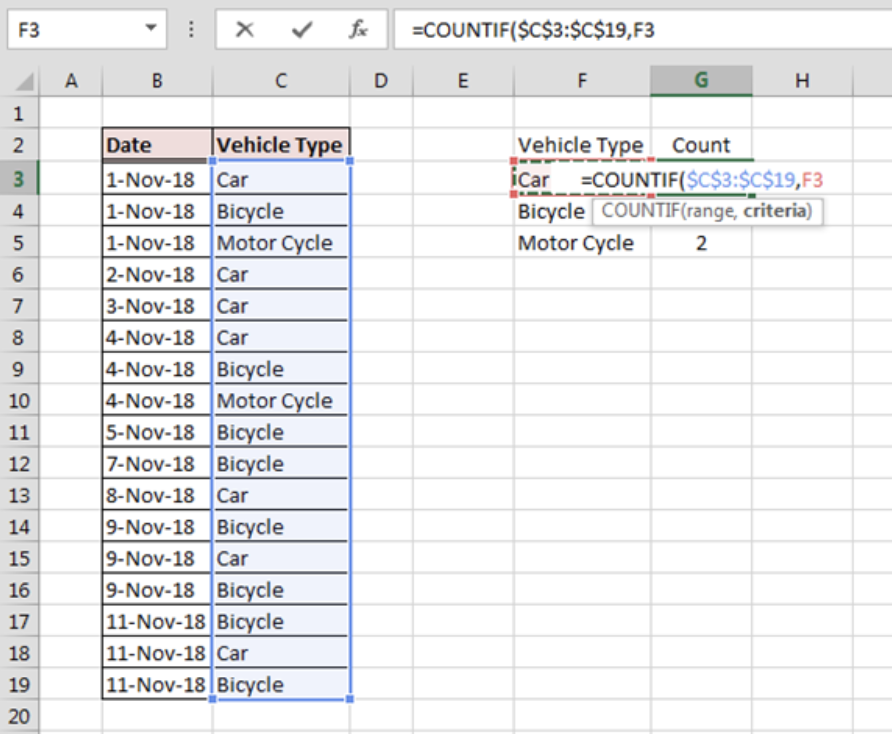Figure 2: Usage of the COUNTIF Formula on the given dataset

• We will calculate the number of vehicles within each type in cells G3 to G5, using the “COUNTIF” formula
• We will break down the COUNTIF formula in cell G3 as below:
`= COUNTIF(\$C\$3:\$C\$19,F3)`
• The range C3:C19 refers to our dataset that elaborates the type of vehicle rented out on a given date
• F3 refers to the vehicle type that we would like to count, “Car” in this case

We can apply the same formula for the “Bicycle” and “Motorcycle” categories in cells G4 and G5 respectively. The results can be seen in the figure below.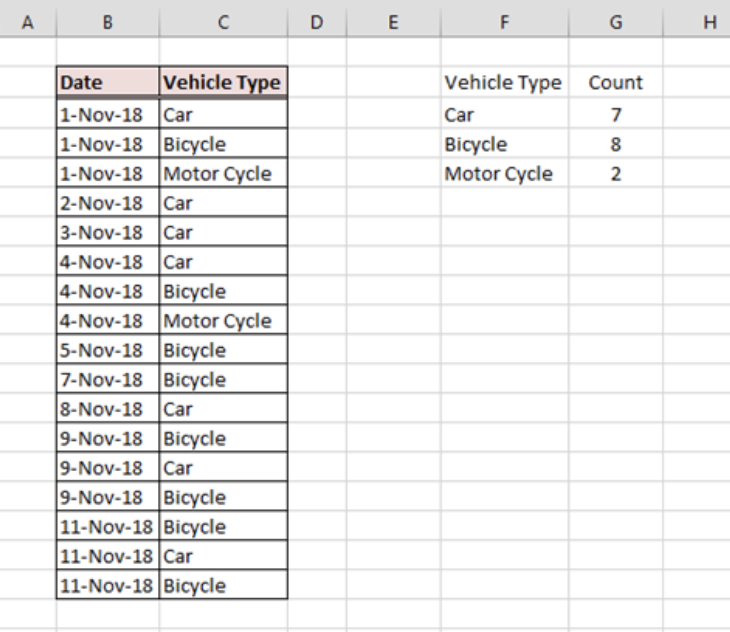Figure 3: COUNTIF result

## Implementation of Conditional Formatting

Now, we will apply conditional formatting to the cells that contain the COUNTIF formula.

Conditional formatting helps us identify the least and most rented vehicle.

The application of the conditional formatting is discussed in the steps below:

• Select cells G3:G5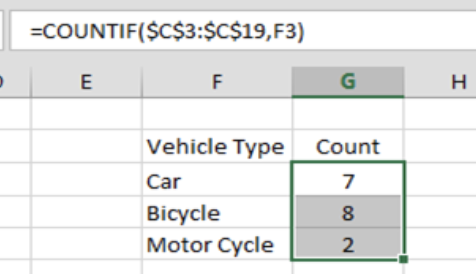Figure 4: Cell Selection for Conditional Formatting

• Go to Home > Conditional Formatting and click the small drop down menu adjacent to conditional formatting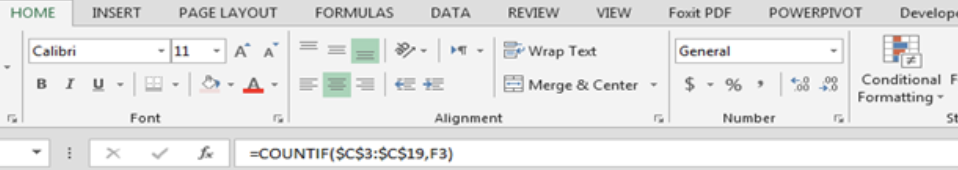Figure 5: Selection of Conditional Formatting from Home

• Select the preferred Color Scale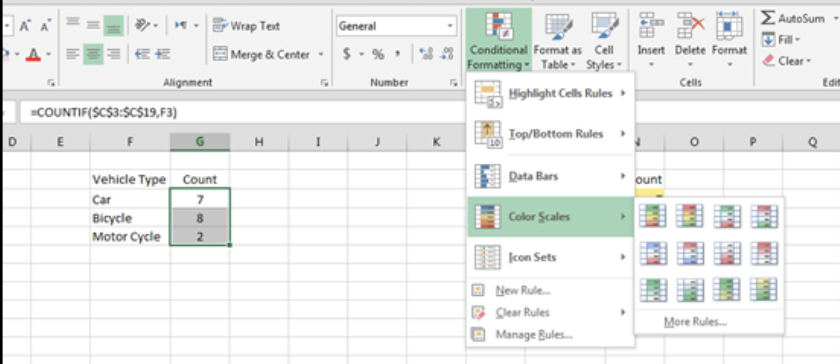Figure 6: Selection of Color Scale

• Select any option in Color Scale and the cells containing the COUNTIF formula will be highlighted based on the selected option.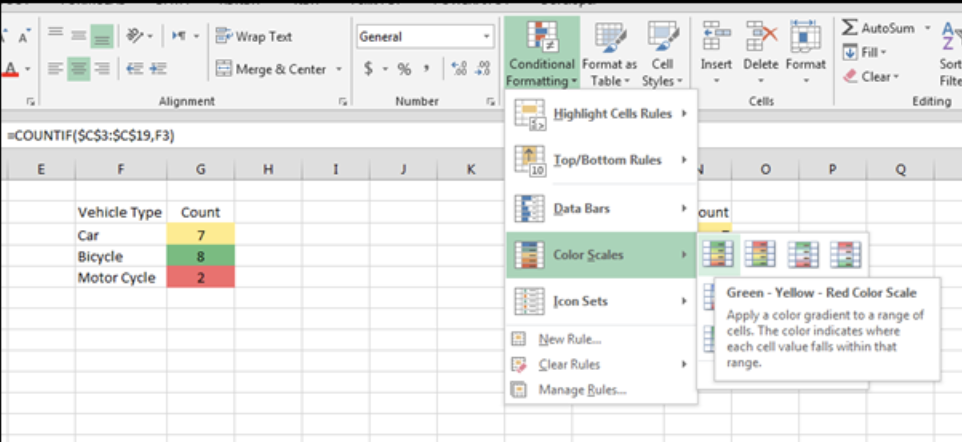Figure 7: Application of Conditional Formatting

Combining Excel functions together can help us solve complex tasks quickly.

The final result will be: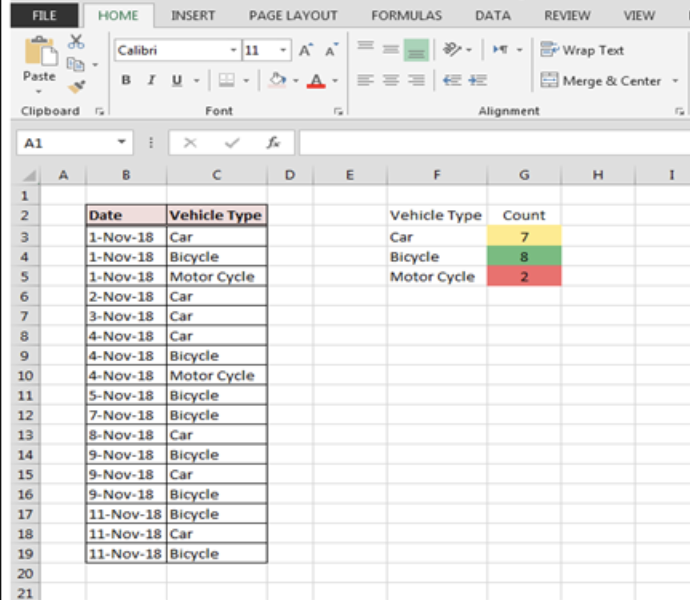Figure 8: Final Result

### Did this post not answer your question? Get a solution from connecting with the expert.Another blog reader asked this question today on Excelchat:
Solution examplesI need a formula to count the number of rows that contain at least one of two possible phrases, but not give me the total number of instances that both phrases occur overall.
Solved by E. B. in 33 minsi have a table with Dates, First names , Last names and i want to count how many times a name occurs, but if a name occurs more than once on a particular date i need to only count it once. this is on excel and not google sheets
Solved by F. H. in 31 minsNeed a formula that counts (1,2,3,4 ect..in cell M21) a range of cells, N4:N20, which are annual premium values. But I only want to add a sale count if the average monthly premium is above \$30 per sale. So if someone has 4 sales but they don't equal a total of \$120/mth (\$30 x 4 = \$120)... they would only get a count of 3 sales.
Solved by S. E. in 60 minsHello, I am trying to create a total color column? Can anyone help?
Solved by I. A. in 60 minsAny rep that has orders over 500 and occurrences at zero for each month, for a 6 month period. I need a sum
Solved by C. E. in 60 mins## Subscribe to Excelchat.coAnother blog reader asked this question today on Excelchat: